## Excel PDURATION Function

The PDURATION function calculates the time or the number of periods required for an investment to reach a specific value at a given rate.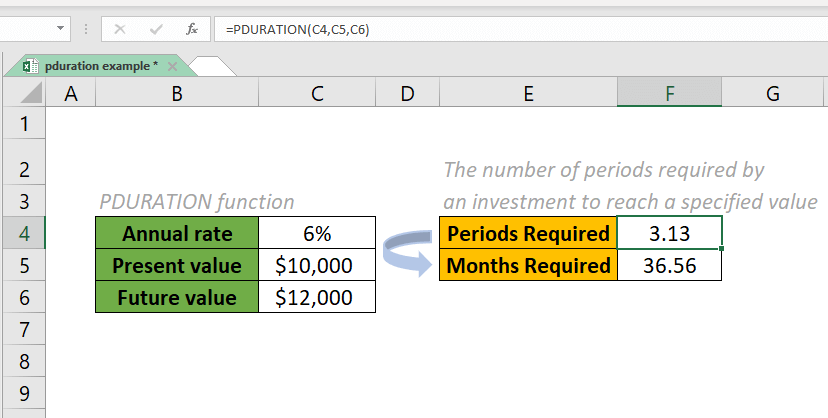#### Syntax

=PDURATION (rate, pv, fv)

#### Arguments

• Rate (required): The interest rate per period.
• Pv (required): The present value of the investment.
• Fv (required): The desired future value of the investment.

√ Note: In most cases, we use the annual interest rate. Monthly and quarterly interest rates are also acceptable.

#### Return value

The PDURATION function returns the required number of periods for an investment to reach a specific value.

#### Function notes

1. Mathematically, the PDURATION function uses the following equation: =(LOG(Fv)-LOG(Pv))/LOG(1+Rate).
2. All arguments must be positive values. Otherwise, the #NUM! error value will be returned.
3. The #VALUE! error value will be returned when any of the supplied arguments use invalid data types.

#### Examples

Suppose we have \$10,000 to invest at an annual rate of 6%. How much time will it take to reach \$12,000? Please see the screenshot.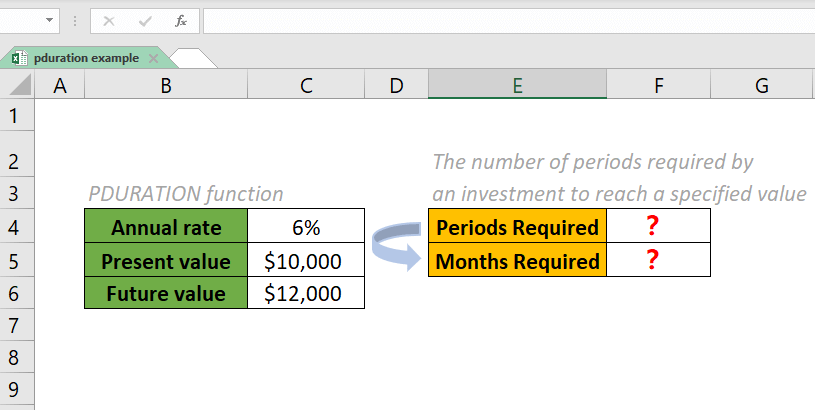In this case, the periods mean "years". To get the result of periods required, please do as follows.

1. Copy the formula below into cell F4 and press the Enter key to get the result.

=PDURATION (C4, C5, C6)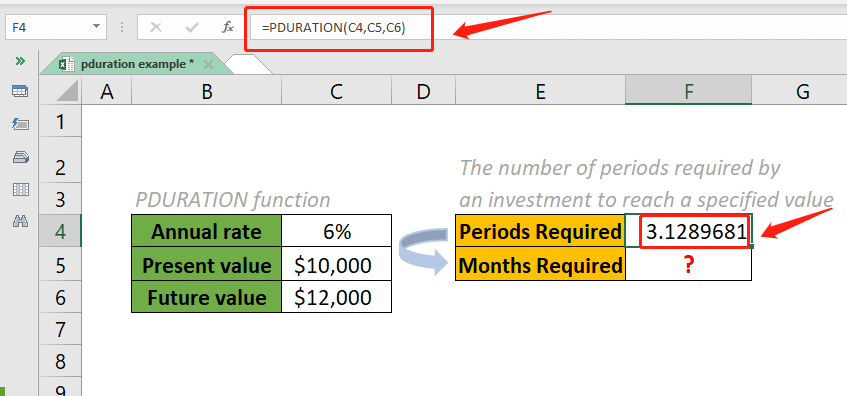2. Round the result to the decimal places we want. Such as two decimal places. Please set up as follows:

• Select the result cell F4 and press CTRL+1. Then a Format Cells dialog box pops up.
• In the Format Cells dialog box, click the tab Number. In the Category list, click Number.
• In the Decimal places box, enter 2 as the number of decimal places that we want to display.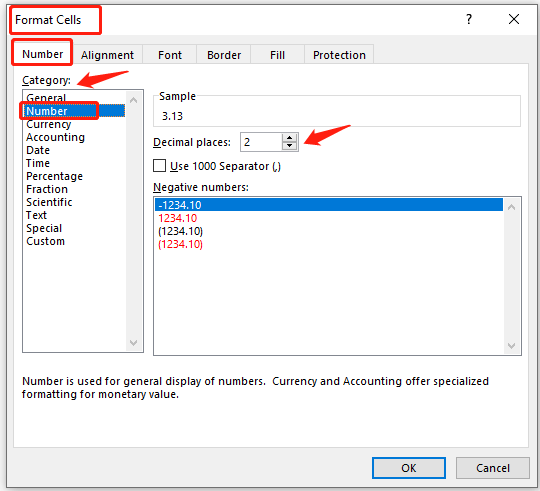3. Click the OK button to get the result.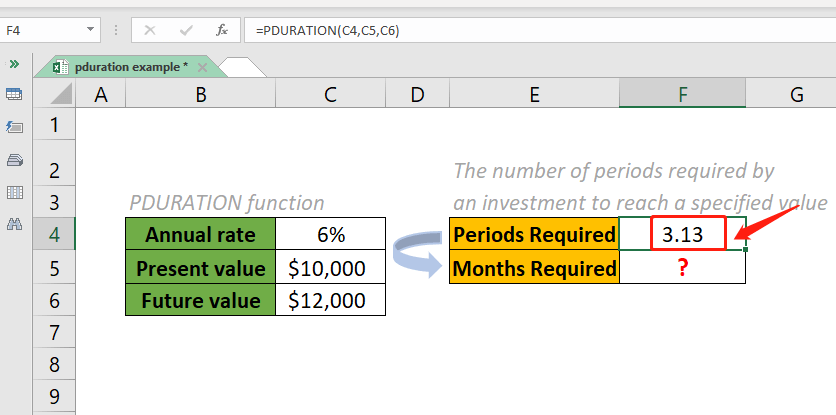As shown in the example above, the annual interest rate is 6%, so the unit of the periods is “years”. What if you want to convert it to “months”? How many months will it take to reach \$12,000? Please do as follows.

1. Copy the formula below into cell F5 and press the Enter key to get the result.

=PDURATION (C4/12, C5, C6)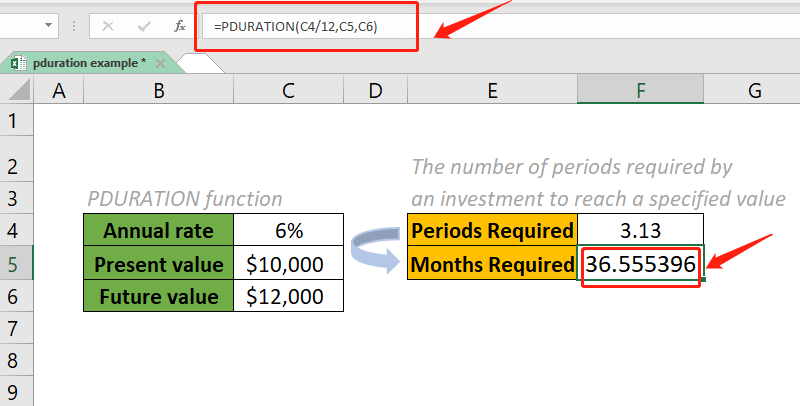2. Round the result to the decimal places we want. Such as two decimal places. Then we get the result.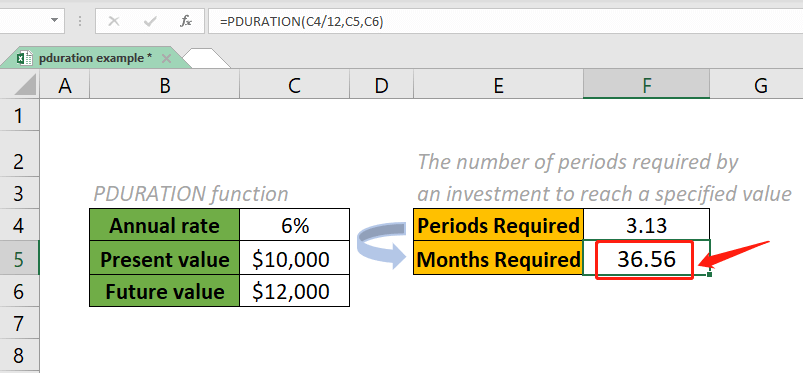Notes:

1. When the periods are equal to months, you should convert the annual rate argument to monthly rate, which is C4/12.
2. We can directly input the values of the three arguments in the formula.
• The formula to get the result of periods (years) required becomes:

=PDURATION (6%, 10000, 12000)

• The formula to get the result of periods (months) required becomes:

=PDURATION (6%/12, 10000, 12000)

#### Relative Functions:

• Excel CUMIPMT Function
The CUMIPMT function returns the cumulative interest paid on a load between the start period and end period.

• Excel CUMPRINC Function
The CUMPRINC function returns the cumulative principal paid on a load between the start period and end period.

### The Best Office Productivity Tools

#### Kutools for Excel - Helps You To Stand Out From Crowd

 Popular Features: Find, Highlight or Identify Duplicates  |  Delete Blank Rows  |  Combine Columns or Cells without Losing Data  |  Round without Formula ... Super VLookup: Multiple Criteria  |  Multiple Value  |  Across Multi-Sheets  |  Fuzzy Lookup... Adv. Drop-down List: Easy Drop Down List  |  Dependent Drop Down List  |  Multi-select Drop Down List... Column Manager: Add a Specific Number of Columns  |  Move Columns  |  Toggle Visibility Status of Hidden Columns  |  Compare Columns to Select Same & Different Cells ... Featured Features: Grid Focus  |  Design View  |  Big Formula Bar  |  Workbook & Sheet Manager | Resource Library (Auto Text)  |  Date Picker  |  Combine Worksheets  |  Encrypt/Decrypt Cells  |  Send Emails by List  |  Super Filter  |  Special Filter (filter bold/italic/strikethrough...) ... Top 15 Toolsets:  12 Text Tools (Add Text, Remove Characters ...)  |  50+ Chart Types (Gantt Chart ...)  |  40+ Practical Formulas (Calculate age based on birthday ...)  |  19 Insertion Tools (Insert QR Code, Insert Picture from Path ...)  |  12 Conversion Tools (Numbers to Words, Currency Conversion ...)  |  7 Merge & Split Tools (Advanced Combine Rows, Split Excel Cells ...)  |  ... and more

Kutools for Excel Boasts Over 300 Features, Ensuring That What You Need is Just A Click Away...#### Office Tab - Enable Tabbed Reading and Editing in Microsoft Office (include Excel)

• One second to switch between dozens of open documents!
• Reduce hundreds of mouse clicks for you every day, say goodbye to mouse hand.
• Increases your productivity by 50% when viewing and editing multiple documents.
• Brings Efficient Tabs to Office (include Excel), Just Like Chrome, Edge and Firefox.